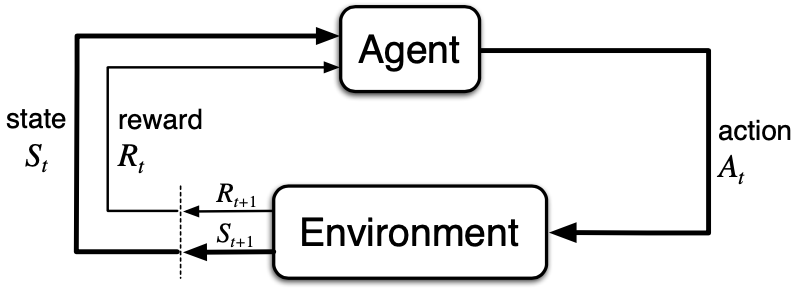# 有限MDP(Markov Decision Process)

这一章将介绍在本书其余部分将要解决的问题。事实上，这个问题定义了强化学习领域：任何适合解决这个问题的方法，我们都可以认为是强化学习方法。

本章的目标是从广义上描述强化学习问题，这包括：描述强化学习问题的数学形式、介绍强化学习问题的关键元素，例如值函数和贝尔曼方程等、讨论强化学习文中的一些权衡和挑战。

首先，我们来分享一些basic的东西，包括关键元素、强化学习问题的简单分类以及符号定义。

#### Basic首先是两个主体：Agent和Environment，两个主体之间的交互关系如图所示。通常Environment决定了我们要解决的问题，Agent就是我们要训练的来解决强化学习问题的主体。两个主体进行交互，是通过动作、状态、奖励实现的。

现在更具体地说说Agent和Environment在离散时间序列中的每一次交互：在每个时间步 t(t = 1,2,\cdots)，Agent接收Environment的状态表示，S_t \in \mathcal{S}，其中 \mathcal{S} 是可能状态的集合，并在此基础上选择一个动作 A_t \in \mathcal{A}(S_t)，其中 \mathcal{A}(S_t) 是状态 S_t 下可采取的动作集合。一个时间步后，Agent收到一个数字奖励，R_{t+1} \in R \subset \mathbb{R}，并处于一个新状态 S_{t+1}

在每个时间步，Agent 维护一个从状态到可能动作的概率的映射。这种映射称为Agent的策略，记为 \pi_t，其中 \pi_t(a|s) 是在 S_t = sA_t = a 的概率。强化学习方法的核心就是指导Agent根据其经验不断地优化自己的策略，Agent的目标就是最大化它在长期内获得的总奖励。

现在，我们来说说强化学习问题的目标和 reward 之间的关系，我们的目标通常都是非形式化的，reward 的设定正是为了将我们的非形式化目标可以直接与强化学习框架结合起来。关于这一点，书中提到了 reward\ hypothesis

That all of what we mean by goals and purposes can be well thought of as the maximization of the expected value of the cu- mulative sum of a received scalar signal (called reward).

基于训练目标的描述，在第 t 个时间步，回报有如下的形式化表示：

G_t = R_{t+1} + R_{t+2} + R_{t+3} + \cdots + R_T,

G_t = R_{t+1} + \gamma R_{t+2} + \gamma^2 R_{t+3} + \cdots = \sum_{k=0}^{\infty}\gamma^k R_{t+k+1}.

此外，强化学习过程还被分为两种不同的形式：有限的和无限的过程。这里为了将两种形式下的优化目标进行统一描述，我们尝试通过设置一个 aborbing\ state 将有限的过程表示为无限的过程，aborbing\ state 事实上就是终止状态，在这个状态之后，所有的奖励值都设置为 0 ，次状态设置为其本身即可。

## 马尔科夫性质

理想情况下，我们想要的是一个可以总结过去所有经验的状态，至少应该保留进行决策所需的所有相关信息。这通常需要的不仅仅是当前时间步的直接感受，还有一些历史信息。成功保留所有相关信息的状态称为马尔可夫的或具有马尔可夫性质。这有时也被称为 independence\ of\ path 属性，因为重要的是当前状态，而已经与历史状态关系不大了，因为当前状态已经包含了我们所需要的所有信息。

为了通过简单的数学形式表示马尔科夫性质，这里假设状态数为有限个。考虑在第 t+1 个时间步，Environment 会对第 t 个时间步的action做出什么样的反馈，这个反馈可能取决于之前发生过的所有的交互。因此，使用一个概率分布来表示这个事情就是

Pr\{R_{t+1}=r,S_{t+1}=s'|S_0,A_0,R_1,\cdots,S_{t-1},A_{t-1},R_t,S_t,A_t\}.

当然，如果状态具有马尔科夫性质，也就是说每个次状态都只与当前状态有关，那我们的状态公式就可以表示为

p(s',r|s,a) = Pr\{R_{t+1}=r,S_{t+1}=s'|S_t,A_t\}.

## Markov Decision Process

一个满足马尔科夫性质的强化学习任务就是 Markov Decision Process或者简写为 MDP，MDP有两个核心的概率公式：一个是 \pi(a|s)，另一个是 p(s',r|s,a)\pi(a|s) 是我们要训练得到的决策函数，p(s',r|s,a) 则主要是由环境决定的，虽然我们直观上想可能觉得在确定了状态以及该状态下的动作之后，次状态和奖励值将会是确定的，然而事实并非如此。因此，在考虑强化学习问题时也要将这个不确定的因素考虑进去。

p(s',r|s,a) 由环境决定，基于这个式子，我们可以推断出很多有用的变量值

r(s,a) = E[R_{t+1}|S_t=s,A_t=a] = \sum_{r\in \mathcal{R}}r\sum_{s'\in\mathcal{S}}p(s',r|s,a),

p(s'|s,a) = Pr\{S_{t+1}=s'|S_t=s,A_t=a\}=\sum_{r\in \mathcal{R}}p(s',r|s,a)

r(s,a,s') = E[R_{t+1}|S_t=s,A_t=a,S_{t+1}=s'] = \frac{\sum_{r\in \mathcal{R}}rp(s',r|s,a)}{p(s'|s,a)}.

## 值函数 Value Function

所有的强化学习任务都需要对值函数进行评估，值函数有两个：状态值函数 v_\pi(s)q_\pi(s,a)v_\pi(s) 是状态值函数，用于评估每个状态的好坏，q_\pi(s,a) 是动作值函数，用于评估每个状态下动作的好坏。先回忆一下之前说过的策略 \pi(a|s)，说的是在状态 s 下采取不同的动作 a 的概率。在每个既定策略下，我们都可以用如下的形式表示值函数

v_{\pi}(s)=\mathbb{E}_{\pi}\left[G_{t} \mid S_{t}=s\right]=\mathbb{E}_{\pi}\left[\sum_{k=0}^{\infty} \gamma^{k} R_{t+k+1} \mid S_{t}=s\right],\\
q_{\pi}(s, a)=\mathbb{E}_{\pi}\left[G_{t} \mid S_{t}=s, A_{t}=a\right]=\mathbb{E}_{\pi}\left[\sum_{k=0}^{\infty} \gamma^{k} R_{t+k+1} \mid S_{t}=s, A_{t}=a\right].

上面说的均值事实上是依托于当前状态与次状态之间的递推关系的，以状态值函数为例，我们有如下推导

\begin{aligned}
v_{\pi}(s) &=\mathbb{E}_{\pi}\left[G_{t} \mid S_{t}=s\right] \\
&=\mathbb{E}_{\pi}\left[\sum_{k=0}^{\infty} \gamma^{k} R_{t+k+1} \mid S_{t}=s\right] \\
&=\mathbb{E}_{\pi}\left[R_{t+1}+\gamma \sum_{k=0}^{\infty} \gamma^{k} R_{t+k+2} \mid S_{t}=s\right]\\
&=\mathbb{E}_{\pi}\left[R_{t+1}\mid S_{t}=s\right]+\gamma\mathbb{E}_{\pi}\left[\sum_{k=0}^{\infty} \gamma^{k} R_{t+k+2} \mid S_{t}=s\right]\\
&=\sum_{a} \pi(a \mid s) \sum_{r} p\left(r \mid s, a\right)r+
\gamma\sum_{a} \pi(a \mid s) \sum_{s^{\prime}} p\left(s^{\prime}\mid s, a\right)\mathbb{E}_{\pi}\left[\sum_{k=0}^{\infty} \gamma^{k} R_{t+k+2} \mid S_{t+1}=s^{\prime}\right]\\
&=\sum_{a} \pi(a \mid s) \sum_{r} \sum_{s^{\prime}} p\left(s^{\prime}, r \mid s, a\right)r+
\gamma\sum_{a} \pi(a \mid s) \sum_{s^{\prime}} \sum_{r} p\left(s^{\prime}, r \mid s, a\right)\mathbb{E}_{\pi}\left[\sum_{k=0}^{\infty} \gamma^{k} R_{t+k+2} \mid S_{t+1}=s^{\prime}\right]\\
&=\sum_{a} \pi(a \mid s) \sum_{s^{\prime}, r} p\left(s^{\prime}, r \mid s, a\right)\left[r+\gamma v_{\pi}\left(s^{\prime}\right)\right]
\end{aligned}

## 最优值函数

是否存在最优值函数呢？如果我们定义了比较两个策略好坏的标准，那么理论上就存在最优策略。我们定义两个策略 \pi\pi'\pi \geq \pi' 当且仅当 \forall s, v_\pi(s) \geq v_{\pi'}(s)。如果 \pi_* 是最优策略，那么就应该有

\forall s,\forall \pi,v_{*}(s) \geq v_{\pi}(s),

\forall s\in\mathcal{S}, v_*(s) = \max_\pi v_\pi(s),

\forall s\in\mathcal{S},\forall a\in \mathcal{A}(s),q_*(s,a) = \max_\pi q_\pi(s,a).

于是，我们可以得到状态值函数和动作值函数之间的递推关系以及当前状态与次状态之间的递推关系，这些递推关系都是在强化学习优化过程中的核心公式：

\begin{aligned}
v_{*}(s) &=\max _{a \in \mathcal{A}(s)} q_{\pi_{*}}(s, a) \\
&=\max _{a} \mathbb{E}_{\pi^{*}}\left[G_{t} \mid S_{t}=s, A_{t}=a\right] \\
&=\max _{a} \mathbb{E}_{\pi^{*}}\left[\sum_{k=0}^{\infty} \gamma^{k} R_{t+k+1} \mid S_{t}=s, A_{t}=a\right] \\
&=\max _{a} \mathbb{E}_{\pi^{*}}\left[R_{t+1}+\gamma \sum_{k=0}^{\infty} \gamma^{k} R_{t+k+2} \mid S_{t}=s, A_{t}=a\right] \\
&=\max _{a} \mathbb{E}\left[R_{t+1}+\gamma v_{*}\left(S_{t+1}\right) \mid S_{t}=s, A_{t}=a\right] \\
&=\max _{a \in \mathcal{A}(s)} \sum_{s^{\prime}, r} p\left(s^{\prime}, r \mid s, a\right)\left[r+\gamma v_{*}\left(s^{\prime}\right)\right] .
\end{aligned}
\begin{aligned}
q_{*}(s, a) &=\mathbb{E}\left[R_{t+1}+\gamma \max _{a^{\prime}} q_{*}\left(S_{t+1}, a^{\prime}\right) \mid S_{t}=s, A_{t}=a\right] \\
&=\sum_{s^{\prime}, r} p\left(s^{\prime}, r \mid s, a\right)\left[r+\gamma \max _{a^{\prime}} q_{*}\left(s^{\prime}, a^{\prime}\right)\right]
\end{aligned}

## 总结

以上就是MDP的基本知识，下次我们分享强化学习的迭代训练方法 -- Dynamic\ Programming